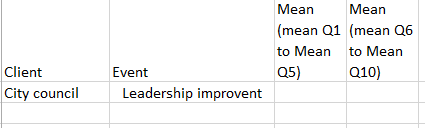# New to Qlik Sense

Discussion board where members can get started with Qlik Sense.

Highlighted
New Contributor III

## How to select some contents from a column and calculate?

Hi dear all, I am trying to help my company build a survey results analysis and now I have some problems.

I have a table below(see attachment)And I would like to generate a table below:Now I was stocked at how to achieve calculating mean (Mean Q1 to Mean Q5), as an measurement. I tried to use do loop function at the expression filed, but it didn't work. Here is what I have tried so far:

Set Q1mean= 0;

Set Q2mean=0;

Set Q3mean=0;

set Q4mean=0;

set Q5mean=0;

Set a=1;

Do while ([Custom workshop.question]='Q1'or [Custom workshop.question]='Q2' or [Custom workshop.question]='Q3' or [Custom workshop.question]='Q4' or [Custom workshop.question]='Q5')

Q1mean=[Custom workshop.question value]+Q1mean;

Q2mean=[Custom workshop.question value]+Q2mean;

Q3mean=[Custom workshop.question value]+Q3mean;

Q4mean=[Custom workshop.question value]+Q4mean;

Q5mean=[Custom workshop.question value]+Q5mean;

Let a=a+1;

Loop

return (Q1mean+Q2mean+Q3mean+Q4mean+Q5mean)/count(distinct Respondent_No)

But it did't work. Anyone could give some suggestions are really appreciated.

Tags (3)
7 Replies
Valued Contributor III

## Re: How to select some contents from a column and calculate?

Dear Rachel Zhong,

Can you share what output are you expecting from the Excel Data?

Thanks,

Mukram.MVP

## Re: How to select some contents from a column and calculate?

The RangeAvg() function could be what you are after - here is the link to its online manual entry which has some examples :

RangeAvg - script and chart function ‒ Qlik Sense

New Contributor III

## Re: How to select some contents from a column and calculate?

Hi Mohammed,

Thanks for your reply, I have attached the table sample I expect to achieve.

Rachel

New Contributor III

## Re: How to select some contents from a column and calculate?

IHi Bill, thanks for your reply. Could you explain more clearly?MVP

## Re: How to select some contents from a column and calculate?

Ignore the RangeAvg() function I wrongly guessed about your input data.

Having seen your xlsx and assuming it is your input data then maybe something like the attached qvf.MVP

## Re: How to select some contents from a column and calculate?

Or if you are after the overall average of the discrete averages then maybe something like this qvf

New Contributor III

## Re: How to select some contents from a column and calculate?

Thank you Bill, it works. I made some changes and got the results. !